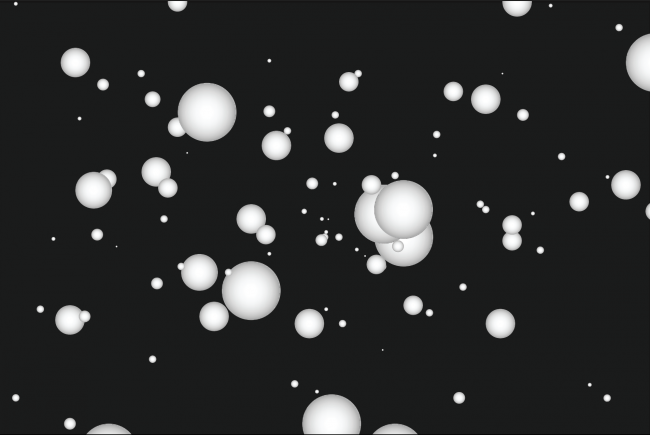#### Howdy, Stranger!

We are about to switch to a new forum software. Until then we have removed the registration on this forum.

# How to make a class of this?

edited June 2016

Hi guys, i'm trying to make a new ball launched after mousePressed, keeping the balls in the secren. I've got this so far, but for some reason it's not working, i was wondering if you could help me.

thanks

``````class Ball {

PVector location;
PVector velocity;
PVector acceleration;
PVector gravity;
PVector lance;
float friction ;
int depth;

Ball(){
depth = 3000;
smooth();
gravity = new PVector(0, 0.98, 0);
location = new PVector(width/2, height/2, -4000);
velocity = new PVector(0, 10, -30);
lance = new PVector(0, 0, 0);
friction = 1.0; //0.98;
}

void update(){

lance = new PVector(0, 0, 0);
velocity.mult(friction);
}

void display(){

noStroke();
fill(255);
lights();
translate(location.x, location.y, location.z);
sphere(35);

if ((location.z < 40)) {
friction = 0.76;
gravity = new PVector(0, 0, 0);
} else {
friction = 1.0;
gravity = new PVector(0, 0.98, 0);
}

}
}

Ball b;

void setup(){

size(800, 800, P3D);
b = new Ball();

}

void draw(){
background(0);
noStroke();
fill(0);
rect(0, 0, width, height);

if (mousePressed) {
location = new PVector(mouseX, mouseY, 300);
lance = new PVector (0, -20, -17);
velocity = new PVector(0, 0, 0);
}

}
``````Tagged:

• You want many objects out of your class? Use Collections! ArrayList is the simple one.

`ArrayList<Ball> balls = new ArrayList<Ball>(); // create arraylist`

``````for (Ball b: balls){
in your mousePressed: `balls.add(new Ball());`